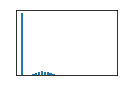# Statistics of neighbors#

When characterizing tissues, it may be useful to summarize statistics such as the average distance labels to their neighbors.

Note: When measuring distances, the centroid-to-centroid distance is called distance for simplicity unless mentioned otherwise. Furthermore, keep in mind that most of the shown measurements only work correctly when using label images with isotropic pixels / voxels.

import numpy as np
import pyclesperanto_prototype as cle
import matplotlib.pyplot as plt
import pandas as pd


We use this example label image to show what the different measurements mean. In the following, most measures will be explained for the object number 7 in the center of the image.

labels = cle.scale(cle.asarray([
[1, 1, 2, 2, 3, 3, 3, 3],
[1, 1, 2, 2, 3, 3, 3, 3],
[1, 1, 7, 7, 7, 7, 3, 3],
[1, 1, 7, 7, 7, 7, 3, 3],
[6, 6, 7, 7, 7, 7, 4, 4],
[6, 6, 7, 7, 7, 7, 4, 4],
[5, 5, 5, 5, 5, 5, 4, 4],
[5, 5, 5, 5, 5, 5, 4, 4],
]), factor_x=10, factor_y=10, auto_size=True).astype(np.uint32)

labels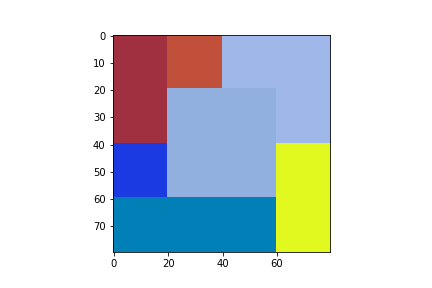cle._ image
 shape (80, 80) dtype uint32 size 25.0 kB min 1.0 max 7.0

# Distance meshes#

Before diving into details we should first have a look at neighborhood relationships and distances between neighbors. A distance mesh visualizes the distances between centroids in colour.

distance_mesh = cle.draw_distance_mesh_between_touching_labels(labels)
cle.imshow(distance_mesh, colorbar=True, colormap="rainbow")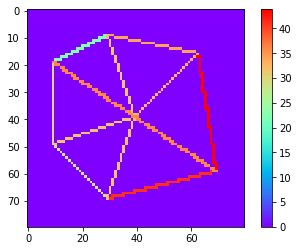Simple statistics such as the longest distance between direct neighbors can be measured from that image.

distance_mesh.max()

43.843155


For more detailed statistics we use a table / pandas DataFrame.

stats = pd.DataFrame(cle.statistics_of_labelled_neighbors(labels))
stats

label touching_neighbor_count minimum_distance_of_touching_neighbors average_distance_of_touching_neighbors maximum_distance_of_touching_neighbors max_min_distance_ratio_of_touching_neighbors proximal_neighbor_count_d10 proximal_neighbor_count_d20 proximal_neighbor_count_d40 proximal_neighbor_count_d80 ... touch_portion_above_0.2_neighbor_count touch_portion_above_0.33_neighbor_count touch_portion_above_0.5_neighbor_count touch_portion_above_0.75_neighbor_count touch_count_sum minimum_touch_count maximum_touch_count minimum_touch_portion maximum_touch_portion standard_deviation_touch_portion
0 1 3.0 22.360680 29.472065 36.055511 1.612452 0.0 0.0 3.0 6.0 ... 3.0 3.0 0.0 0.0 60.0 20.0 20.0 0.333333 0.333333 2.980232e-08
1 2 3.0 22.360680 29.325642 33.993465 1.520234 0.0 0.0 3.0 6.0 ... 3.0 3.0 0.0 0.0 60.0 20.0 20.0 0.333333 0.333333 2.980232e-08
2 3 3.0 32.998318 36.944984 43.843155 1.328648 0.0 0.0 2.0 6.0 ... 3.0 2.0 0.0 0.0 80.0 20.0 40.0 0.250000 0.500000 1.111111e-01
3 4 3.0 36.055511 40.376575 43.843155 1.215990 0.0 0.0 1.0 6.0 ... 3.0 2.0 0.0 0.0 60.0 20.0 20.0 0.333333 0.333333 2.980232e-08
4 5 3.0 28.284273 33.712704 41.231056 1.457738 0.0 0.0 2.0 6.0 ... 3.0 2.0 0.0 0.0 80.0 20.0 40.0 0.250000 0.500000 1.111111e-01
5 6 3.0 28.284273 29.969015 31.622776 1.118034 0.0 0.0 3.0 6.0 ... 3.0 2.0 0.0 0.0 60.0 20.0 20.0 0.333333 0.333333 2.980232e-08
6 7 6.0 31.622776 33.329613 36.055511 1.140175 0.0 0.0 6.0 6.0 ... 6.0 6.0 0.0 0.0 160.0 20.0 40.0 0.125000 0.250000 5.555556e-02

7 rows × 44 columns

This table contains these columns:

stats.describe().T

count mean std min 25% 50% 75% max
label 7.0 4.000000 2.160247 1.000000e+00 2.500000e+00 4.000000e+00 5.500000 7.000000
touching_neighbor_count 7.0 3.428571 1.133893 3.000000e+00 3.000000e+00 3.000000e+00 3.000000 6.000000
minimum_distance_of_touching_neighbors 7.0 28.852358 5.190999 2.236068e+01 2.532248e+01 2.828427e+01 32.310547 36.055511
average_distance_of_touching_neighbors 7.0 33.304371 4.184873 2.932564e+01 2.972054e+01 3.332961e+01 35.328844 40.376575
maximum_distance_of_touching_neighbors 7.0 38.092091 4.881063 3.162278e+01 3.502449e+01 3.605551e+01 42.537106 43.843155
max_min_distance_ratio_of_touching_neighbors 7.0 1.341896 0.193760 1.118034e+00 1.178083e+00 1.328648e+00 1.488986 1.612452
proximal_neighbor_count_d10 7.0 0.000000 0.000000 0.000000e+00 0.000000e+00 0.000000e+00 0.000000 0.000000
proximal_neighbor_count_d20 7.0 0.000000 0.000000 0.000000e+00 0.000000e+00 0.000000e+00 0.000000 0.000000
proximal_neighbor_count_d40 7.0 2.857143 1.573592 1.000000e+00 2.000000e+00 3.000000e+00 3.000000 6.000000
proximal_neighbor_count_d80 7.0 6.000000 0.000000 6.000000e+00 6.000000e+00 6.000000e+00 6.000000 6.000000
proximal_neighbor_count_d160 7.0 6.000000 0.000000 6.000000e+00 6.000000e+00 6.000000e+00 6.000000 6.000000
maximum_distance_of_n1_nearest_neighbors 7.0 28.852358 5.191000 2.236068e+01 2.532248e+01 2.828427e+01 32.310547 36.055511
average_distance_of_n1_nearest_neighbors 7.0 28.852358 5.191000 2.236068e+01 2.532248e+01 2.828427e+01 32.310547 36.055511
maximum_distance_of_n2_nearest_neighbors 7.0 32.870403 3.922370 3.000000e+01 3.081139e+01 3.162278e+01 32.808121 41.231056
average_distance_of_n2_nearest_neighbors 7.0 30.861380 4.257244 2.618034e+01 2.806693e+01 2.995353e+01 32.559334 38.643284
maximum_distance_of_n3_nearest_neighbors 7.0 37.458839 5.444354 3.162278e+01 3.280812e+01 3.605551e+01 42.537104 43.843155
average_distance_of_n3_nearest_neighbors 7.0 33.060535 4.232608 2.932564e+01 2.972054e+01 3.162278e+01 35.328842 40.376572
maximum_distance_of_n4_nearest_neighbors 7.0 49.142155 9.086052 3.299832e+01 4.472136e+01 5.343740e+01 53.644524 60.827621
average_distance_of_n4_nearest_neighbors 7.0 37.080940 4.918169 3.196666e+01 3.341583e+01 3.546340e+01 39.907761 45.489334
maximum_distance_of_n5_nearest_neighbors 7.0 56.808464 9.705204 3.605551e+01 5.692582e+01 6.000000e+01 61.860416 64.031242
average_distance_of_n5_nearest_neighbors 7.0 41.026447 5.346182 3.278444e+01 3.881543e+01 3.914105e+01 44.215530 49.197720
maximum_distance_of_n6_nearest_neighbors 7.0 61.855495 12.137788 3.605551e+01 6.289321e+01 6.289321e+01 68.071133 72.111023
average_distance_of_n6_nearest_neighbors 7.0 44.497955 6.060761 3.332961e+01 4.292323e+01 4.463604e+01 47.328476 53.016602
maximum_distance_of_n7_nearest_neighbors 7.0 61.855495 12.137788 3.605551e+01 6.289321e+01 6.289321e+01 68.071133 72.111023
average_distance_of_n7_nearest_neighbors 7.0 48.585533 5.194938 3.901267e+01 4.723577e+01 4.870390e+01 51.011694 55.887230
maximum_distance_of_n8_nearest_neighbors 7.0 61.855495 12.137788 3.605551e+01 6.289321e+01 6.289321e+01 68.071133 72.111023
average_distance_of_n8_nearest_neighbors 7.0 48.585533 5.194938 3.901267e+01 4.723577e+01 4.870390e+01 51.011694 55.887230
maximum_distance_of_n10_nearest_neighbors 7.0 61.855495 12.137788 3.605551e+01 6.289321e+01 6.289321e+01 68.071133 72.111023
average_distance_of_n10_nearest_neighbors 7.0 48.585533 5.194938 3.901267e+01 4.723577e+01 4.870390e+01 51.011694 55.887230
maximum_distance_of_n20_nearest_neighbors 7.0 61.855495 12.137788 3.605551e+01 6.289321e+01 6.289321e+01 68.071133 72.111023
average_distance_of_n20_nearest_neighbors 7.0 48.585533 5.194938 3.901267e+01 4.723577e+01 4.870390e+01 51.011694 55.887230
distance_to_most_distant_other 7.0 61.855495 12.137788 3.605551e+01 6.289321e+01 6.289321e+01 68.071133 72.111023
touch_portion_above_0_neighbor_count 7.0 3.428571 1.133893 3.000000e+00 3.000000e+00 3.000000e+00 3.000000 6.000000
touch_portion_above_0.16_neighbor_count 7.0 3.428571 1.133893 3.000000e+00 3.000000e+00 3.000000e+00 3.000000 6.000000
touch_portion_above_0.2_neighbor_count 7.0 3.428571 1.133893 3.000000e+00 3.000000e+00 3.000000e+00 3.000000 6.000000
touch_portion_above_0.33_neighbor_count 7.0 2.857143 1.463850 2.000000e+00 2.000000e+00 2.000000e+00 3.000000 6.000000
touch_portion_above_0.5_neighbor_count 7.0 0.000000 0.000000 0.000000e+00 0.000000e+00 0.000000e+00 0.000000 0.000000
touch_portion_above_0.75_neighbor_count 7.0 0.000000 0.000000 0.000000e+00 0.000000e+00 0.000000e+00 0.000000 0.000000
touch_count_sum 7.0 80.000000 36.514839 6.000000e+01 6.000000e+01 6.000000e+01 80.000000 160.000000
minimum_touch_count 7.0 20.000000 0.000000 2.000000e+01 2.000000e+01 2.000000e+01 20.000000 20.000000
maximum_touch_count 7.0 28.571428 10.690450 2.000000e+01 2.000000e+01 2.000000e+01 40.000000 40.000000
minimum_touch_portion 7.0 0.279762 0.078743 1.250000e-01 2.500000e-01 3.333333e-01 0.333333 0.333333
maximum_touch_portion 7.0 0.369048 0.094491 2.500000e-01 3.333333e-01 3.333333e-01 0.416667 0.500000
standard_deviation_touch_portion 7.0 0.039683 0.052844 2.980232e-08 2.980232e-08 2.980232e-08 0.083333 0.111111

The following code snippets show how we can interpret that table. All examples refer to the labeled object 7 in the center of the label image shown above.

print("The label of the last object is",
stats["label"].tolist()[-1])

The label of the last object is 7

print("The last object has",
stats["touching_neighbor_count"].tolist()[-1],
"touching neighbors")

The last object has 6.0 touching neighbors

print("The maximum distance of any label centroid to any other is",
stats["maximum_distance_of_touching_neighbors"].max())

The maximum distance of any label centroid to any other is 43.843155

print("The last object has an average distance to its touching neighbors of",
stats["average_distance_of_touching_neighbors"].tolist()[-1]
)

The last object has an average distance to its touching neighbors of 33.329612731933594

print("The last object has an minimum distance to its touching neighbors of",
stats["minimum_distance_of_touching_neighbors"].tolist()[-1]
)

The last object has an minimum distance to its touching neighbors of 31.62277603149414

print("The last object has an maximum distance to its touching neighbors of",
stats["maximum_distance_of_touching_neighbors"].tolist()[-1]
)

The last object has an maximum distance to its touching neighbors of 36.055511474609375

for d in [10, 20, 40, 80]:
print("There are",
stats["proximal_neighbor_count_d" + str(d)].tolist()[-1],
"objects around the last labeled object within a radius of",
d,
"pixels"
)

There are 0.0 objects around the last labeled object within a radius of 10 pixels
There are 0.0 objects around the last labeled object within a radius of 20 pixels
There are 6.0 objects around the last labeled object within a radius of 40 pixels
There are 6.0 objects around the last labeled object within a radius of 80 pixels

for n in [1,2,3,4,5,6]:
print("The " + str(n) + ". neighbor of the last label is",
stats["maximum_distance_of_n" + str(n) + "_nearest_neighbors"].tolist()[-1],
"pixels away."
)

The 1. neighbor of the last label is 31.62277603149414 pixels away.
The 2. neighbor of the last label is 31.62277603149414 pixels away.
The 3. neighbor of the last label is 31.62277603149414 pixels away.
The 4. neighbor of the last label is 32.99831771850586 pixels away.
The 5. neighbor of the last label is 36.055511474609375 pixels away.
The 6. neighbor of the last label is 36.055511474609375 pixels away.

for n in [1,2,3,4,5,6]:
print("The average distance to the " + str(n) + " neighbors of the last label is",
stats["average_distance_of_n" + str(n) + "_nearest_neighbors"].tolist()[-1],
"pixels."
)

The average distance to the 1 neighbors of the last label is 31.62277603149414 pixels.
The average distance to the 2 neighbors of the last label is 31.62277603149414 pixels.
The average distance to the 3 neighbors of the last label is 31.622777938842773 pixels.
The average distance to the 4 neighbors of the last label is 31.966663360595703 pixels.
The average distance to the 5 neighbors of the last label is 32.7844352722168 pixels.
The average distance to the 6 neighbors of the last label is 33.329612731933594 pixels.


## Tocuh count and touch portion#

Just for reader’s convenience we visualize the label image again.

labelscle._ image
 shape (80, 80) dtype uint32 size 25.0 kB min 1.0 max 7.0
print("The last labelled object has",
stats["touch_count_sum"].tolist()[-1],
"pixels where it touches others")

The last labelled object has 160.0 pixels where it touches others

print("The last labelled object touches an other object with at least",
stats["minimum_touch_count"].tolist()[-1],
"pixels")

The last labelled object touches an other object with at least 20.0 pixels

print("The last labelled object touches an other object with up to",
stats["maximum_touch_count"].tolist()[-1],
"pixels")

The last labelled object touches an other object with up to 40.0 pixels

print("The last labelled object touches an other object with at least",
stats["minimum_touch_portion"].tolist()[-1] * 100,
"percent of its border")

The last labelled object touches an other object with at least 12.5 percent of its border

print("The last labelled object touches an other object with up to",
stats["maximum_touch_portion"].tolist()[-1] * 100,
"percent of its border")

The last labelled object touches an other object with up to 25.0 percent of its border


## Visualization of statistics#

We can visualize those measurements in parametric map images.

For visualization of the table columns as maps, we typically need to prefix the measurements with a 0. This 0 represents the measurement of the background.

stats["touching_neighbor_count"].tolist()

[3.0, 3.0, 3.0, 3.0, 3.0, 3.0, 6.0]

list_of_measurements = cle.prefix_in_x([stats["touching_neighbor_count"].tolist()])
list_of_measurements

cle.array([[0. 3. 3. 3. 3. 3. 3. 6.]], dtype=float32)
cle.replace_intensities(labels, list_of_measurements)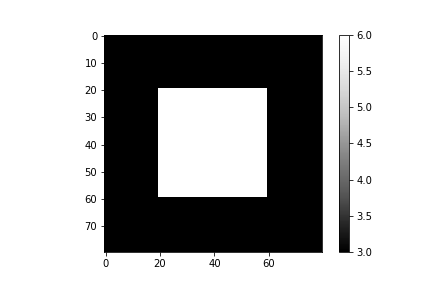cle._ image
 shape (80, 80) dtype float32 size 25.0 kB min 3.0 max 6.0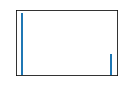As more realistic example, we visualize the measurements in an image stack of a Tribolium embryo. The dataset, curtesy of Daniela Vorkel / Myers lab / MPI-CBG / CSBD can be downloaded here.

embryo = imread("../../../clesperanto_example_data/Lund-100MB.tif")

nuclei_labels = cle.voronoi_otsu_labeling(bg_subtracted, spot_sigma=0.5, outline_sigma=1)


fig, axs = plt.subplots(1, 4, figsize=(10,10))

axs.set_title("Raw")
cle.imshow(embryo, plot=axs)
axs.set_title("Background subtracted")
cle.imshow(bg_subtracted, plot=axs)
axs.set_title("Nuclei segmentation")
cle.imshow(nuclei_labels, plot=axs, labels=True)
axs.set_title("Cell estimation")
cle.imshow(cell_estimation, plot=axs, labels=True)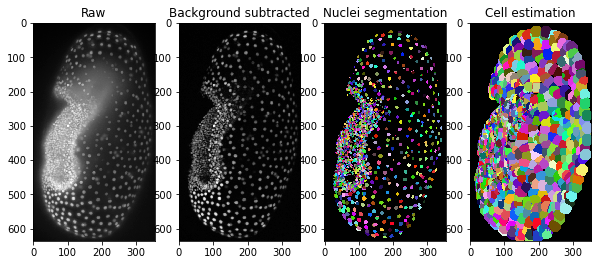tribolium_statistics = pd.DataFrame(cle.statistics_of_labelled_neighbors(cell_estimation))

label touching_neighbor_count minimum_distance_of_touching_neighbors average_distance_of_touching_neighbors maximum_distance_of_touching_neighbors proximal_neighbor_count_d10 proximal_neighbor_count_d20 proximal_neighbor_count_d40 proximal_neighbor_count_d80 proximal_neighbor_count_d160 ... average_distance_of_n10_nearest_neighbors maximum_distance_of_n20_nearest_neighbors average_distance_of_n20_nearest_neighbors distance_to_most_distant_other touch_count_sum minimum_touch_count maximum_touch_count minimum_touch_portion maximum_touch_portion standard_deviation_touch_portion
0 1 11.0 10.348568 15.763863 25.492878 0.0 9.0 42.0 195.0 665.0 ... 15.164477 27.046114 20.066113 482.901642 1586.0 12.0 373.0 0.007566 0.235183 0.065240
1 2 4.0 9.680110 11.968576 15.206567 1.0 5.0 26.0 149.0 568.0 ... 19.315979 35.638351 25.482178 505.435364 1077.0 38.0 486.0 0.035283 0.451253 0.117224
2 3 7.0 7.612343 13.007790 15.458411 1.0 7.0 44.0 201.0 690.0 ... 16.436604 28.811810 21.466221 473.300323 632.0 4.0 308.0 0.006329 0.487342 0.100555
3 4 4.0 9.382964 11.711300 13.744253 1.0 9.0 39.0 190.0 648.0 ... 15.705339 28.550455 20.369110 486.618164 584.0 48.0 214.0 0.082192 0.366438 0.085616
4 5 8.0 9.382964 13.527851 17.916573 1.0 8.0 37.0 180.0 620.0 ... 15.669672 29.883175 21.231649 494.449829 1499.0 15.0 420.0 0.010007 0.280187 0.067357

5 rows × 37 columns

def visualize(label_image, statistics, column):
list_of_measurements = cle.prefix_in_x([statistics[column].tolist()])

return cle.replace_intensities(label_image, list_of_measurements)

visualize(cell_estimation, tribolium_statistics, "label")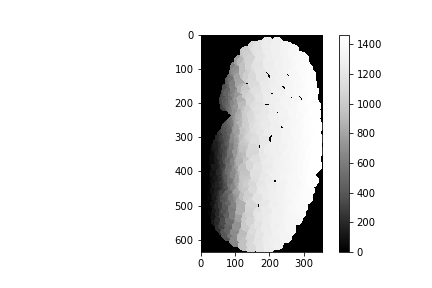cle._ image
 shape (116, 636, 354) dtype float32 size 99.6 MB min 0.0 max 1465.0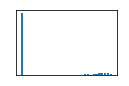### Number of touching neighbors and proximal neighbors#

visualize(cell_estimation, tribolium_statistics, "touching_neighbor_count")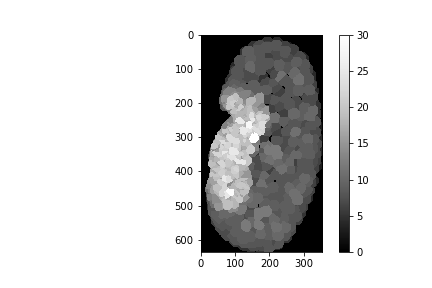cle._ image
 shape (116, 636, 354) dtype float32 size 99.6 MB min 0.0 max 30.0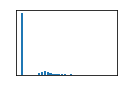visualize(cell_estimation, tribolium_statistics, "proximal_neighbor_count_d10")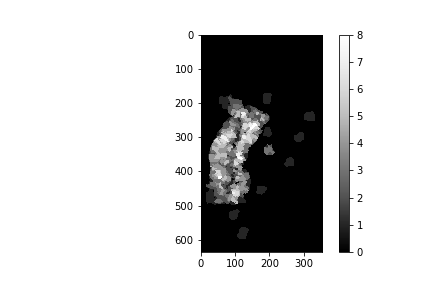cle._ image
 shape (116, 636, 354) dtype float32 size 99.6 MB min 0.0 max 8.0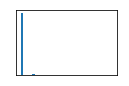visualize(cell_estimation, tribolium_statistics, "proximal_neighbor_count_d20")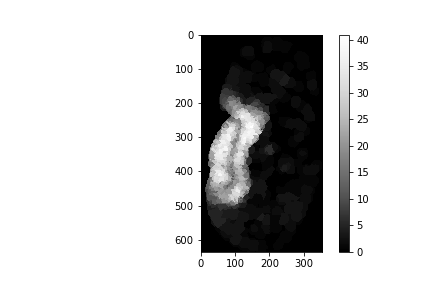cle._ image
 shape (116, 636, 354) dtype float32 size 99.6 MB min 0.0 max 41.0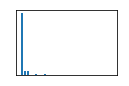visualize(cell_estimation, tribolium_statistics, "proximal_neighbor_count_d40")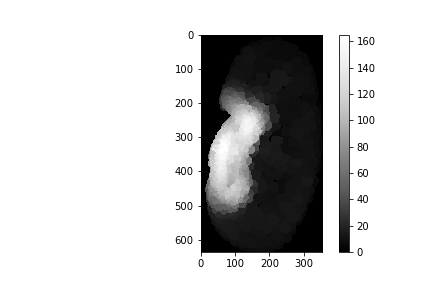cle._ image
 shape (116, 636, 354) dtype float32 size 99.6 MB min 0.0 max 165.0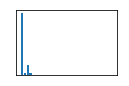### Distances to touching neighbors#

visualize(cell_estimation, tribolium_statistics, "minimum_distance_of_touching_neighbors")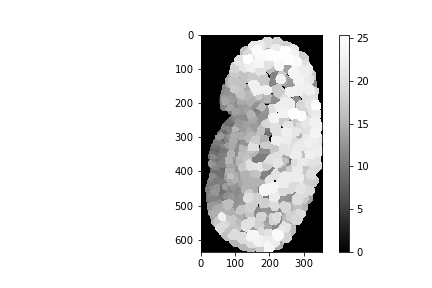cle._ image
 shape (116, 636, 354) dtype float32 size 99.6 MB min 0.0 max 25.412409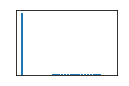visualize(cell_estimation, tribolium_statistics, "average_distance_of_touching_neighbors")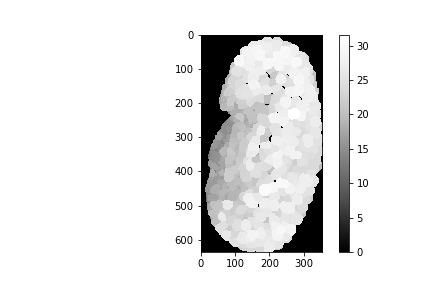cle._ image
 shape (116, 636, 354) dtype float32 size 99.6 MB min 0.0 max 31.604395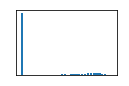visualize(cell_estimation, tribolium_statistics, "maximum_distance_of_touching_neighbors")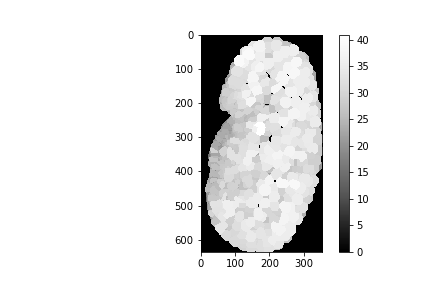cle._ image
 shape (116, 636, 354) dtype float32 size 99.6 MB min 0.0 max 41.0165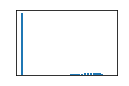### Distance to nearest neighbors#

visualize(cell_estimation, tribolium_statistics, "maximum_distance_of_n1_nearest_neighbors")cle._ image
 shape (116, 636, 354) dtype float32 size 99.6 MB min 0.0 max 25.412409visualize(cell_estimation, tribolium_statistics, "maximum_distance_of_n6_nearest_neighbors")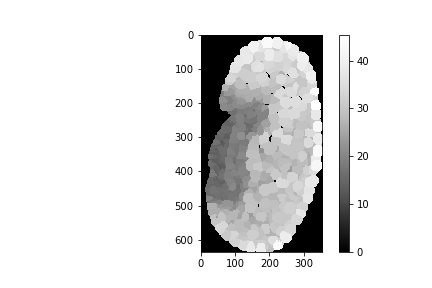cle._ image
 shape (116, 636, 354) dtype float32 size 99.6 MB min 0.0 max 45.45253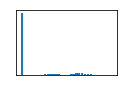visualize(cell_estimation, tribolium_statistics, "maximum_distance_of_n10_nearest_neighbors")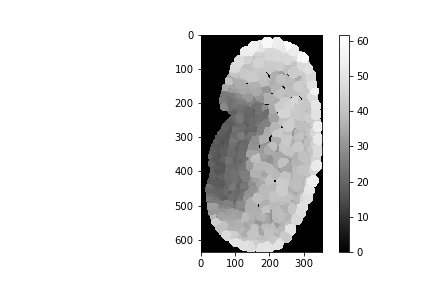cle._ image
 shape (116, 636, 354) dtype float32 size 99.6 MB min 0.0 max 61.80885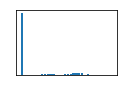### Distance to the most distant other label#

visualize(cell_estimation, tribolium_statistics, "distance_to_most_distant_other")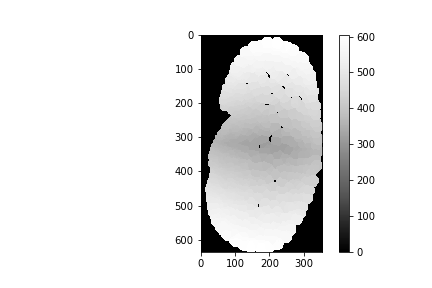cle._ image
 shape (116, 636, 354) dtype float32 size 99.6 MB min 0.0 max 605.44824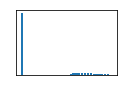### Touch count#

Touch count is the number of voxels labels touch others.

visualize(cell_estimation, tribolium_statistics, "touch_count_sum")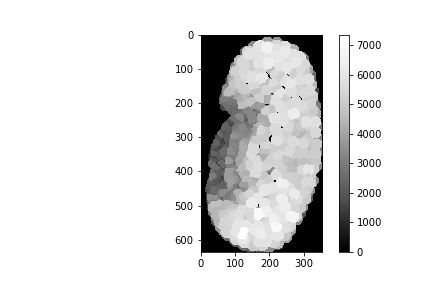cle._ image
 shape (116, 636, 354) dtype float32 size 99.6 MB min 0.0 max 7360.0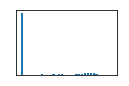visualize(cell_estimation, tribolium_statistics, "minimum_touch_count")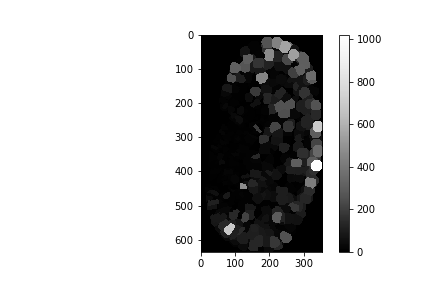cle._ image
 shape (116, 636, 354) dtype float32 size 99.6 MB min 0.0 max 1023.0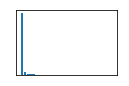visualize(cell_estimation, tribolium_statistics, "maximum_touch_count")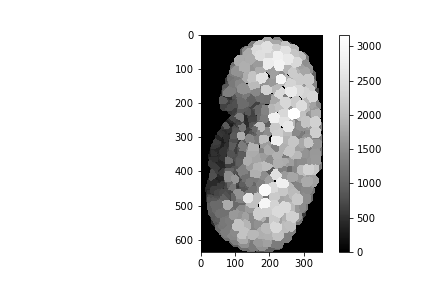cle._ image
 shape (116, 636, 354) dtype float32 size 99.6 MB min 0.0 max 3172.0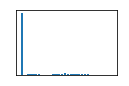### Touch portion#

Touch portion is the relative amount of pixels where objects touch one other object divided by the number of all pixels where the object touches others.

visualize(cell_estimation, tribolium_statistics, "minimum_touch_portion")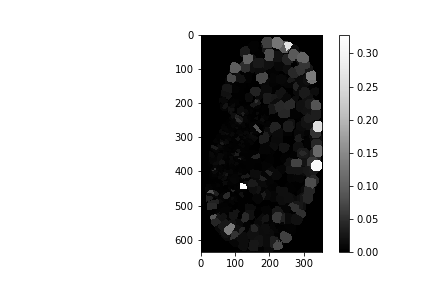cle._ image
 shape (116, 636, 354) dtype float32 size 99.6 MB min 0.0 max 0.3285124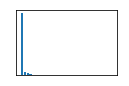visualize(cell_estimation, tribolium_statistics, "maximum_touch_portion")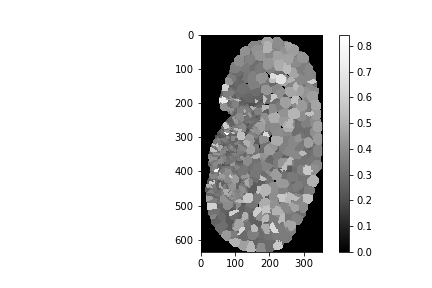cle._ image
 shape (116, 636, 354) dtype float32 size 99.6 MB min 0.0 max 0.84375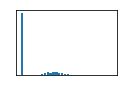visualize(cell_estimation, tribolium_statistics, "standard_deviation_touch_portion")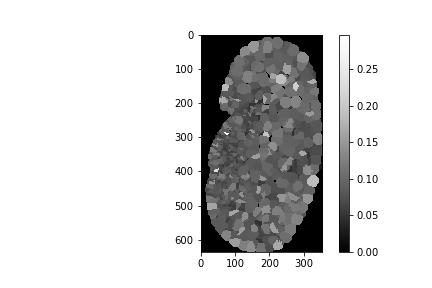cle._ image
 shape (116, 636, 354) dtype float32 size 99.6 MB min 0.0 max 0.296875